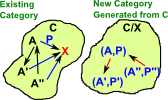# Category Theory - Slice Category Examples

Slice category is a specific case of comma category.

## Slice Category

Given a category C we can 'slice' it over some object XC which we fix in C.

 This construction allows us to start with one category 'C' and generate a different category 'C/X' by fixing a given element 'X' in C. The elements in C/X are pairs (A,P) where AC and P is a morphism from A to X.#### Slice Category Examples

 Colouring of labeled set. Here we choose as our fixed object 'X' the 3 element set containing R, G and B (for red green and blue). Every object (in this case set) has an arrow (function) to this set so all the elements are assigned a colour. When we add functions between these set objects these functions must now commute. This restricts us to functions which only map R to R, G to G and B to B. This effectively partitions the structure into 3 separate sets, one for each colour. This seems similar to fibrations. Strictly its only a fibration if the category supports pullbacks. See nlab for explanation of this.In category theory we are not really supposed to look inside objects or arrows, but we do so here to attempt to give some intuition. Adding a distinguished point (the origin) to Euclidean space to give a vector space.'co-slice' is the dual concept, reverse all the arrows.

### Comma Category References

Where I can, I have put links to Amazon for books that are relevant to the subject, click on the appropriate country flag to get more details of the book or to buy it from them.The Princeton Companion to Mathematics - This is a big book that attempts to give a wide overview of the whole of mathematics, inevitably there are many things missing, but it gives a good insight into the history, concepts, branches, theorems and wider perspective of mathematics. It is well written and, if you are interested in maths, this is the type of book where you can open a page at random and find something interesting to read. To some extent it can be used as a reference book, although it doesn't have tables of formula for trig functions and so on, but where it is most useful is when you want to read about various topics to find out which topics are interesting and relevant to you.